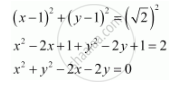Share

# Find the Equation of the Circle with Centre (1, 1) and Radius Sqrt2 - Mathematics

#### Question

Find the equation of the circle with centre (1, 1) and radius sqrt2

#### Solution

The equation of a circle with centre (hk) and radius is given as

(x­ – h)2 + (y ­– k)2 = r2

It is given that centre (hk) = (1, 1) and radius (r) =sqrt2

Therefore, the equation of the circle isIs there an error in this question or solution?
Solution Find the Equation of the Circle with Centre (1, 1) and Radius Sqrt2 Concept: Concept of Circle.